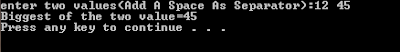# C Program To Find Greatest of the Two Number, Without Using If Else, With Ternary Operator "?"

/*In the following simple C program the user will be asked for 2 numbers, then the ternary operation (a>b)?a:b will calculate the greatest of the two entered numbers. The Bold text is the C Code here, & the comments are in normal font*/

#include<stdio.h>     //For Standard Input/Output Functions
#include<stdlib.h>   //For system("pause")
int main()
{
int a,b,c;
printf("enter two values(Add A Space As Separator):");
scanf("%d%d",&a,&b);
//Entering the 2 values
c=(a>b)?a:b;                      //Calculation of the greatest of the 2 values
printf("Biggest of the two value=%d\n",c); //Printing the biggest of the 2 values
system("pause");
return 0;
}

ScreenShot:C Program To Find Greatest of the Two Number, Without Using If Else, With Ternary Operator "?"